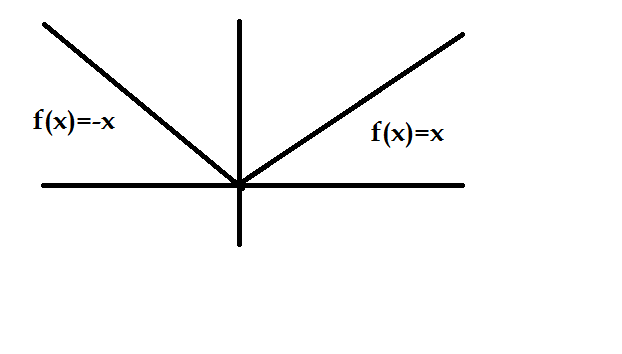# Engineering Maths - Calculus

>>>>>>>>Calculus

• A

-5• B

0• C

-1• D

-2• Option : B
• Explanation : Since value of a mod function cannot be less than zero, therefore f(x) = | x2 - 5x + 21 | is zero.

• A

1/4• B

0• C

-1/4• D

1• A

-2ln2• B

2• C

0• D

(ln2)2• A

minimum at x = 1• B

maximum at x = 1• C

minimum at x = 2• D

maximum at x = 2• Option : A
• Explanation : f(x) = 3x (x - 2) = 3x- 6x Differentiating, we getAgain diferentiating,For maxima or minima, - f '(x) =0 ∴  6x-6=0  ⇒ x=1 As f '(x) =6 >0 then f(x) is minimum at x=1

• A

1• B

-1• C

0• D

Does not exist• Option : D
• Explanation : f(x) = |x| and f(x) = x for x > 0 ⇒f(x) = -x for x < 0Hence from the graph it is clear that derivative doesn't exists for x=0
Related Quiz.
Calculus